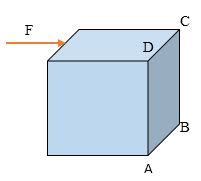A force is applied perpendicular to the edge of a cube of side length 'a' as shown in the diagram.The torque about edge $CD$ and $AB$ respectively is